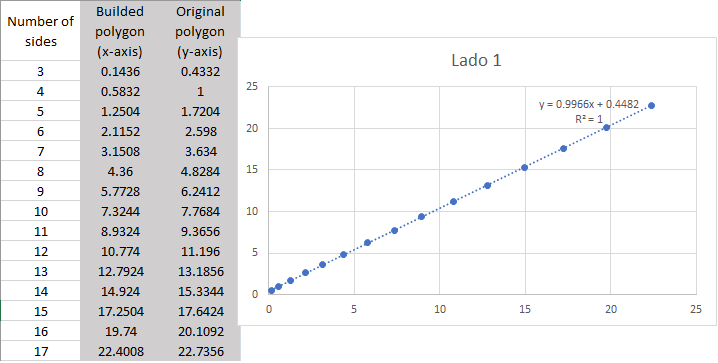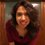# Areas related with a linear equation

Some years ago, I posted two geometrical problems related with side-ratios in triangles and squares. This year, I was on my bed thinking when I remember this two problems and I try to generalize for regular polygons of n-sides. After some calculations in Geogebra, I found an interesting relation between the regular polygon (the original one) and the builded polygon which was a linear equation. The question from which the problem emerges is:

Let be a polygon of n sides (original) with vertex labeled from $A$ to $N$. Between $AB$ there is a point $a$ such $Aa=aB$, between $BC$ there is a point $b$ such $Bb=\frac{1}{3}BC$, between $CD$ there is a point $c$ such $Cc=\frac{1}{4}CD$ and so on until complete the n vertex (builded).

Then, polygons' areas are plotted (axis-$x$ for the area of builded polygons and axis-$y$ for the area of the original polygons) to values from $n=1$ to $n=17$

A linear equation is got it from the previous question.

For polygons of side one I got the areas in te table below which its graphI did the same process for polygons of sides $2$ to $7$ and get the same linear equation. I'm intrigued about:

• Why this data shows a linear equation?

• How can I get a matemathical process to calculate the builded area?

• Why the linear equations is so close from $y=x$?

Those are my main questions, I hope someone get interested in this problem and together we could work together on this.Note by Paola Ramírez
2 years, 7 months ago

This discussion board is a place to discuss our Daily Challenges and the math and science related to those challenges. Explanations are more than just a solution — they should explain the steps and thinking strategies that you used to obtain the solution. Comments should further the discussion of math and science.

When posting on Brilliant:

• Use the emojis to react to an explanation, whether you're congratulating a job well done , or just really confused .
• Ask specific questions about the challenge or the steps in somebody's explanation. Well-posed questions can add a lot to the discussion, but posting "I don't understand!" doesn't help anyone.
• Try to contribute something new to the discussion, whether it is an extension, generalization or other idea related to the challenge.

MarkdownAppears as
*italics* or _italics_ italics
**bold** or __bold__ bold
- bulleted- list
• bulleted
• list
1. numbered2. list
1. numbered
2. list
Note: you must add a full line of space before and after lists for them to show up correctly
paragraph 1paragraph 2

paragraph 1

paragraph 2

[example link](https://brilliant.org)example link
> This is a quote
This is a quote
    # I indented these lines
# 4 spaces, and now they show
# up as a code block.

print "hello world"
# I indented these lines
# 4 spaces, and now they show
# up as a code block.

print "hello world"
MathAppears as
Remember to wrap math in $$ ... $$ or $ ... $ to ensure proper formatting.
2 \times 3 $2 \times 3$
2^{34} $2^{34}$
a_{i-1} $a_{i-1}$
\frac{2}{3} $\frac{2}{3}$
\sqrt{2} $\sqrt{2}$
\sum_{i=1}^3 $\sum_{i=1}^3$
\sin \theta $\sin \theta$
\boxed{123} $\boxed{123}$

Sort by:

- 1 year, 6 months ago

Paola Ramirez posted this 1 year and 1 month ago.

- 1 year, 6 months ago

Yeah, I understand and I think she is not probably active on brilliant right now

- 1 year, 6 months ago

I agree with you. vasu paliwal, when did you start solving problems in brilliant? Are you new here?

- 1 year, 6 months ago